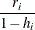# The HPREG Procedure

### Diagnostic Statistics

This section gathers the formulas for the statistics available in the OUTPUT statement. All the statistics available in the OUTPUT statement are conditional on the selected model and do not take into account the variability introduced by doing model selection.

The model to be fit is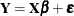, and the parameter estimate is denoted by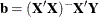. The subscript i denotes values for the ith observation, and the parenthetical subscript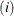means that the statistic is computed by using all observations except the ith observation.

The ALPHA= option in the PROC HPREG statement is used to set thevalue for the confidence limit statistics.

Table 60.6 contains the diagnostic statistics and their formulas. Each statistic is computed for each observation.

Table 60.6: Formulas and Definitions for Diagnostic Statistics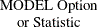Formula

PRED ()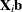RES (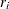)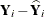H (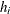)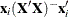STDP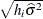STDI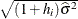STDR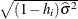LCL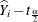STDI

LCLMSTDP

UCL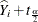STDI

UCLMSTDP

STUDENT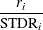RSTUDENT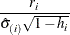COOKD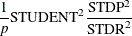COVRATIO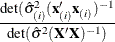DFFITS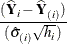PRESS(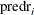)